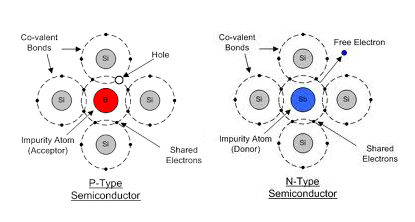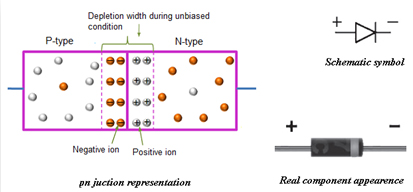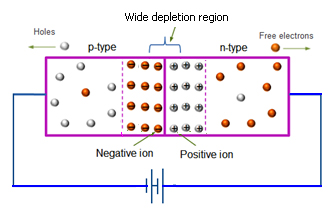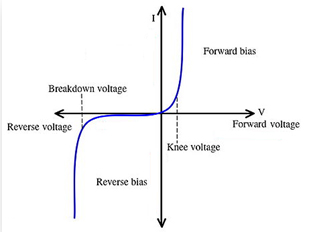Diode characteristics

# Objective

To draw the I-V characteristic curve of a p-n junction diode in forward bias and reverse bias.

# Theory

## Semiconductors

Semiconductors are materials with electrical conductivity intermediate between that of a conductor and an insulator. In semiconducting materials thermal energy is enough to cause a number of electrons to release from valance band to conduction band, in which they are relatively free. Common semiconducting materials are silicon, germanium, gallium, arsenide etc.

Semiconductors are classified as;

Intrinsic semiconductors :Pure semiconducting materials like crystalline form of germanium and silicon, with equal concentration of electrons and holes.

Extrinsic semiconductors :Semiconducting material with the addition of suitable impurity atoms through doping.

Extrinsic semiconductors can be p-type or n-type depending on the impurities added to it. A p- type semiconductor is formed when adding pentavalent impurities like phosphorus, arsenic, antimony etc. to an intrinsic semiconductor. If the impurities added are trivalent atoms, we get the n- type semiconductor.Semiconductor Diodes

Semiconductor diode is simply the combination of a p-type and an n-type material. It is formed by doping half of the silicon crystal with trivalent impurity (p-type) and the other half with pentavalent impurity (n-type). It has the characteristics of passing current in one direction only. If there is no voltage is applied across the junction, electrons will diffuse through the junction to p - side and holes will diffuse through the junction to n - side and they combine with each other. Thus the acceptor atom near the p - side and donor atom near n – side are left unutilized and is called the depletion layer. An electron field is generated by these uncovered charges which called the barrier potential. This opposes further diffusion of carriers and is known as depletion region.Biasing in diodes

Biasing in general means the application of dc voltage across the terminals of a device for a particular operation. Two types of biasing are possible in a p-n junction diode. They are;

### Forward Biasing :Forward biasing occurs when the positive end of the diode is connected to the positive terminal of the battery, and its negative end to the negative terminal of the battery. Here, majority carriers from both sides move towards and cross the junction and current flows through the junction. This current is known as the forward current and is the order of 10-3 A. The size of the depletion layer decreases in forward biasing.

### Reverse Biasing :Reverse biasing occurs when the positive end of the diode is connected to the negative terminal of the battery, and its negative end to the positive terminal of the battery. Here, majority carriers from both sides move away from the junction and thus no current flows through the junction. A very small current will made at the junction due to the movement of minority charge carriers across the junction.

Characteristics of a p-n junction diode

It generally shows the relation between bias voltage and current of a diode. The V-I characteristics of a diode can be forward or reverse. The graph showing the forward bias voltage and forward current is known as the forward characteristics, and that showing the reverse bias voltage and reverse current is known as the reverse characteristics.The forward characteristics of a diode is non linear. The forward current increases slowly in the beginning and shows a sudden rise at a certain value of forward voltage. This voltage is known as the threshold voltage or Knee voltage. This is because the resistance is very low in forward biased condition. The current in the reverse bias is due to the flow of minority carriers. The reverse current shows a sudden increase at a particular region. The corresponding voltage is termed as the reverse breakdown voltage.

# Learning Outcomes

• Students understand p-n junction diodes and their working.
• Students learn the different types of biasing in diodes.
• Students understand the concept of diode characteristics.
• Students learn the different terms associated with p-n junction diodes.